# MATH 251: Calculus 3, SET8

## 16: Vector Calculus

### 16.9: The Divergence Theorem

These problems are done with the CAS. See Hand Solutions for details.

#### 1. [1145/2]

We use the Divergence Theorem to compute the relevant sum of surface integrals by computing a triple integral over the solid.
(See the Hand Solutions for the tedious surface integral computations.) Remember your JacFacs in triple integrals!
syms r x y z theta
F = [y^2*z^3 2*y*z 4*z^2], div_F = divergence(F, [x y z]) % from 16.5
F =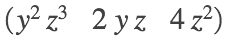div_F =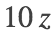I = int(int(int(div_F * r, z, r^2, 9), r, 0, 3), theta, 0, 2*pi)
I =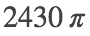format bank
I_appx = double(I)
I_appx = 7634.07
format short
%
figure
fsurf(r*cos(theta), r*sin(theta), r^2, [0 3 0 2*pi], 'g', 'MeshDensity', 12); hold on
fsurf(r*cos(theta), r*sin(theta), 9, [0 3 0 2*pi], 'r', 'MeshDensity', 12)
axis equal; axis([-3 3 -3 3 0 9])
xticks(-3:3:3); yticks(-3:3:3); zticks(0:3:9)
xlabel('x'); ylabel('y'); zlabel('z')
title('SET8, 1145/2')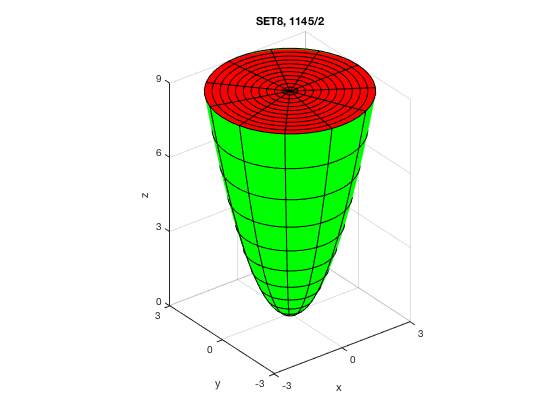#### 2. [1145/4]

Same drill as #1.
syms r x y z theta
F = [x^2 -y z], div_F = divergence(F, [x y z]) % from 16.5
F =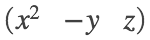div_F =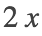I = int(int(int(div_F * r, x, 0, 2), r, 0, 3), theta, 0, 2*pi)
I =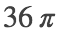I_appx = double(I)
I_appx = 113.0973
%
figure % The x-axis is stretched to better visualize the cylinder and disks.
fsurf(x, 3*cos(theta), 3*sin(theta), [0 2*pi 0 2], 'y', 'EdgeColor', 'none'); hold on
fsurf(2, r*cos(theta), r*sin(theta), [0 3 0 2*pi], 'r', 'EdgeColor', 'none')
fsurf(0, r*cos(theta), r*sin(theta), [0 3 0 2*pi], 'c', 'EdgeColor', 'none')
axis([0 2 -3 3 -3 3])
xticks(0:2); yticks(-3:3:3); zticks(-3:3:3)
xlabel('x'); ylabel('y'); zlabel('z')
title('SET8, 1145/4'); alpha 0.3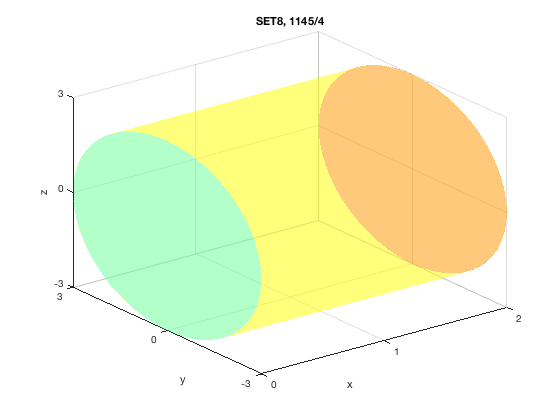#### 3. [1145/6]

As before, we apply the Divergence Theorem to compute the flux through the closed surface, a rectangular box.
syms a b c x y z
F = [x^2*y*z x*y^2*z x*y*z^2], div_F = divergence(F, [x y z]) % from 16.5
F =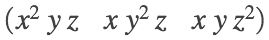div_F =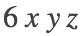I = int(int(int(div_F, x, 0, a), y, 0, b), z, 0, c)
I =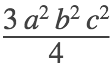#### 4. [1145/8]

We apply the Divergence Theorem to compute the flux through a sphere.
syms x y z rho phi theta
F = [x^3+y^3 y^3+z^3 x^3+z^3], div_F = divergence(F, [x y z]) % from 16.5
F =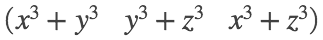div_F =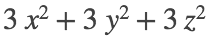div_F = 3*rho^2 % Convert to spherical coordinates.
div_F =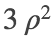I = int(int(int(div_F * rho^2*sin(phi), rho, 0, 2), phi, 0, pi), theta, 0, 2*pi)
I =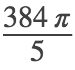I_appx = double(I)
I_appx = 241.2743

#### 5. [1145/10]

We apply the Divergence Theorem to compute the flux through a tetrahedron.
syms a b c x y z
F = [z y x*z], div_F = divergence(F, [x y z]) % from 16.5
F =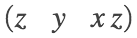div_F =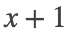I = int(int(int(div_F, z, 0, c*(1 - x/a - y/b)), y, 0, b*(1 - x/a)), x, 0, a)
I =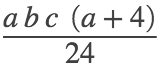#### 6. [1145/12]

We apply the Divergence Theorem to compute the flux through a surface bounded by a circular cylinder and two disks, one circular and one elliptical.
syms r u x y z theta
F = [x*y+2*x*z x^2+y^2 x*y-z^2], div_F = divergence(F, [x y z]) % from 16.5
F =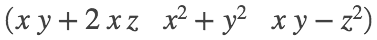div_F =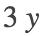div_F = 3*r*sin(theta) % Convert to cylindrical coordinates.
div_F =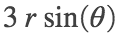I = int(int(int(div_F * r, z, r*sin(theta)-2, 0), r, 0, 2), theta, 0, 2*pi)
I =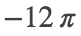I_appx = double(I)
I_appx = -37.6991
%
figure % The x-axis is stretched to better visualize the cylinder and disks.
fsurf(2*cos(theta), 2*sin(theta), u*(2*sin(theta)-2), [0 2*pi 0 1], 'y', 'EdgeColor', 'none')
hold on
fsurf(r*cos(theta), r*sin(theta), r*sin(theta)-2, [0 2 0 2*pi], 'r', 'EdgeColor', 'none')
fsurf(r*cos(theta), r*sin(theta), 0, [0 2 0 2*pi], 'g', 'EdgeColor', 'none')
axis([-2 2 -2 2 -4 0])
xticks(-2:2:2); yticks(-2:2:2); zticks(-4:2:0)
xlabel('x'); ylabel('y'); zlabel('z')
title('SET8, 1145/12'); view(54,12); alpha 0.3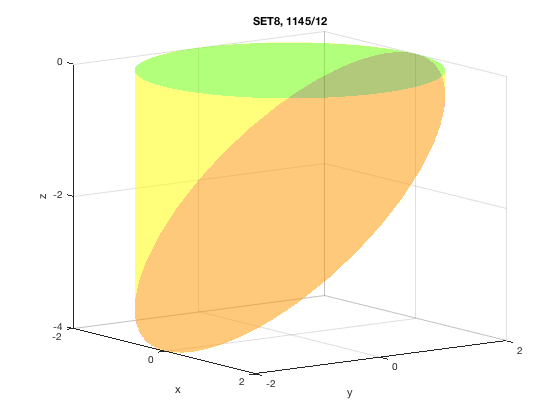#### 7. [1146/14]

We apply the Divergence Theorem to compute the flux through a sphere.
syms a x y z rho phi theta
F = (x^2 + y^2 + z^2)*[x y z],
F =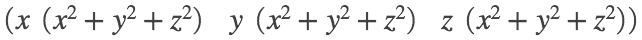div_F = simplify( divergence(F, [x y z]) ) % from 16.5
div_F =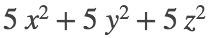div_F = 5*rho^2 % Convert to spherical coordinates.
div_F =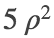I = int(int(int(div_F * rho^2*sin(phi), rho, 0, a), phi, 0, pi), theta, 0, 2*pi)
I =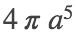#### 8. [1146/16]

We apply the Divergence Theorem to compute the flux through a cube.
syms a b c x y z
F = [sin(x) * cos(y)^2 sin(y)^3 * cos(z)^4 sin(z)^5 * cos(x)^6]
F =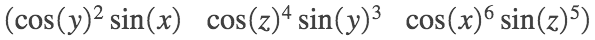div_F = divergence(F, [x y z]) % from 16.5
div_F =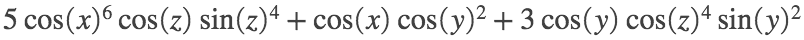I = int(int(int(div_F, x, 0, pi/2), y, 0, pi/2), z, 0, pi/2)
I =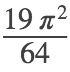I_appx = double(I)
I_appx = 2.9300

#### 9. [1146/18]

We apply the Divergence Theorem to compute the flux through a solid bounded by a paraboloid and a circular disk.
Then we subtract off the flux through the bottom circular disk. This leaves the flux through the paraboloid.
For disk, let r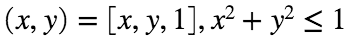. Then F(r(x,y))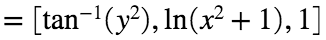. The outward (downward) pointing normal is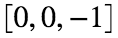. So the flux is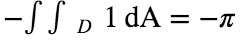.
syms r x y z theta
F = [z*atan(y^2) z^3*log(x^2+1) z], div_F = divergence(F, [x y z]) % from 16.5
F =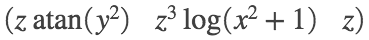div_F = 1
I = int(int(int(div_F * r, z, 1, 2-r^2), r, 0, 1), theta, 0, 2*pi)
I =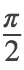I_appx = double(I)
I_appx = 1.5708
%
flux_thru_disk = -sym(pi)
flux_thru_disk =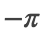flux_thru_paraboloid = I - (-pi)
flux_thru_paraboloid =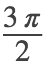%
figure
fsurf(r*cos(theta), r*sin(theta), 2-r^2, [0 1 0 2*pi], 'y', 'MeshDensity', 10); hold on
fsurf(r*cos(theta), r*sin(theta), 1, [0 1 0 2*pi], 'm', 'MeshDensity', 10)
axis equal; axis([-1 1 -1 1 0 3])
xticks(-1:1); yticks(-1:1); zticks(0:3)
xlabel('x'); ylabel('y'); zlabel('z')
title('SET8, 1146/18'); alpha 0.5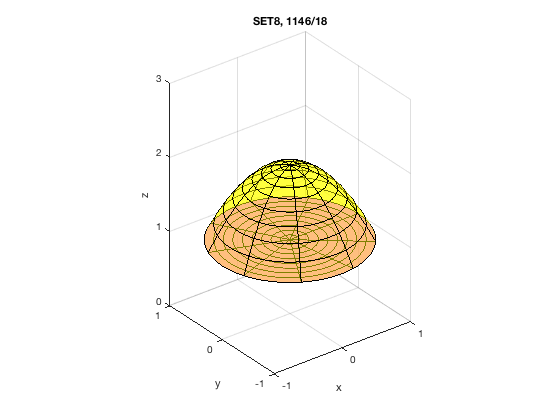#### 10. [1146/24]

An outward pointing normal vector to the sphere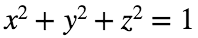is n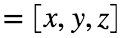. Let F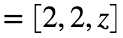. Then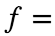F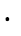n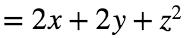, the scalar field stated in the problem. So the surface integral of the scalar field f over the sphere equals the surface integral of the vector field F over the sphere, which we now compute by applying the Divergence Theorem. Since div F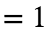, the integral is the volume of a sphere of radius 1, easily computed using multiplication.
syms x y z
F = [2 2 z], div_F = divergence(F, [x y z])
F =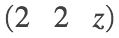div_F = 1
V = sym(4/3 * pi * 1^3) % V = 4/3 * pi * radius^3
V =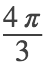V_appx = double(V)
V_appx = 4.1888